6. A nonlinear oscillator.Consider a mass m that is suspended in a spring, where the restoring force F is supposed to bein the distance y from the equilibrium point (with minus sign because the force is opposed to the direction of motion). Newton’s second law then gives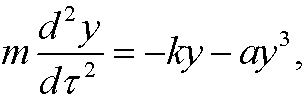with the initial conditions (the mass is dropped from a positive displacement A)We rescale this problem according to(see chapter 1) and get the equationwhereis a dimensionless parameter. This is Duffing’s equation. We try to solve it with our perturbation method. Insert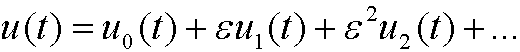in the rescaled differential equation:Now compare powers of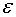: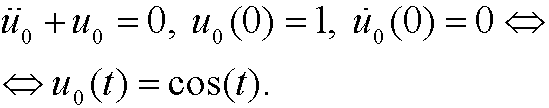An approximate solution is thusNote that:

(i) the leading term cos(t) seems correct.

(ii) if t<=T0 andis “small” then the correction term is “small”.

(iii) if we let t to be large (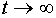) , the correction term can be large even thoughis small.

Remark: The problem in (iii) is due to the secular termOur next move will be to introduce a method that avoids this problem.